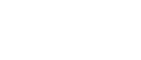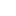#• PRODUCTS• SOLUTIONS• TUTORIALS• SUPPORT• ABOUT AKMGlobal | EnglishChoose your country or region
• Japan
• Global
• United States
• Europe
• China
• Korea
• Taiwan
• India

#• PRODUCTS
• SOLUTIONS
• TUTORIALS
• SUPPORT
• ABOUT AKM

# Building magnetic encoder with high resolution by combining an SMR element and an ADC!

Episode 12. Introducing the AK924X series, new "ZDS-NS Type ADCs" developed by AKM.

#12 Basic Knowledge of ADCThis is a story that young A, who works in a fictional motor company deepens the knowledge of ADCs with a senior colleague K, and his boss, M, manager.

Young A

I heard that the SMR element of AKM is amazing, but what is SMR in the first place?

Expert M Manager

SMR is an abbreviation for, "Semiconductor Magneto-Resistive", meaning semiconductor magnetoresistive effect. The change in the electric resistance value according to the applied magnetic field is called the magnetoresistance effect, and the product applying this effect is the MR element. If you aim for high resolution with a magnetic encoder, you must check AKM's SMR element!

Young A

What is great about AKM's SMR elements?

Expert M Manager

For example, AMR and GMR elements, which are representative MR elements, use ferromagnetic metals. Magnetic hysteresis occurs in the ferromagnetic body due to the magnetic field in principle, so there is a difference in the output voltage depending on the direction of the magnetic field. Since the SMR element is a semiconductor, there is no magnetic hysteresis. This is a big difference.

Young A

Well, magnetic hysteresis is troubling.

I remember it was troublesome even with the current sensor.

Senior K

AKM's SMR elements have extremely good temperature characteristics among SMR elements, and feature exceptionally small element variation.

Young A

Now I know there are also some kinds in SMR elements. Why can it be applied to encoders?

Senior K

When combining a gear wheel and a bias magnet, the output waveform will change according to the teeth and pitch spaces of the gear wheel. Therefore, it can detect the tooth position, that is, the rotation angle. (Figure 1)Figure 1. Rotation Angle Detection with SMR Element

Young A

I see, you use it in combination with a gear wheel.

Senior K

Furthermore, since the output waveform is a sinusoidal wave with less distortion, it can improve resolution with electric interpolation using an ADC. Combined with a high-resolution ADC, it is possible to achieve 20 bits or more resolution in rotation angle detection even with a magnetic encoder!

Young A

20-bits with magnetic encoder! What? Magnetic type is excellent in environmental resistance such as dust, dust, oil, but it is common sense that the resolution cannot be so high.

Expert M Manager

The point is to earn the resolution equivalent to the number of teeth of the gear. For example, when the SMR element detects a 128-tooth gear, the A-phase and the B-phase for one wave are output from the SMR element for each tooth. That is 128 waves per rotation.

Young A

Well, there is also 7-bit resolution with a gear wheel. The number of teeth of the gear wheel corresponds to the slit resolution of the optical encoder. What is the recommended ADC for electric interpolation?

Senior K

The AK924X series is perfect. Since the output amplitude of the SMR element is around 100 mV, amplification is necessary, and the SMR element is, "resistance", as its name implies, so the power to drive the subsequent circuit is weak. Therefore, a high impedance input like an instrumentation amplifier is desirable. (Figure 2)Figure 2. Block Diagram of SMR Element + AK9242

Young A

Moreover, if using the AK9242/43, angle calculation function is built in! I am happy to work less with digital circuits.

Expert M Manager

By the way, the AK9242 is a 16-bit ADC, but in angle calculation mode it has a specification of 18-bit output. What is the reason for this?

Young A

That is true. In angle calculation mode, the output is extended 2 bits. Does the ADC become an 18-bit device in angle calculation mode?

Senior K

The ADC is 16-bit, but has a loss to the output the angle calculation result using 16-bit data in 16-bit.

Young A

Huh? Why?

Senior K

Let's think about what happens to the angular calculation result with a 3-bit ADC to make it easy.

Young A

If the output is 3-bit, you can express up to 8 patterns per rotation, that is, you can represent a minimum of 45 degrees.

Senior K

What happens when the unit circle, that is, the Lissajous waveform with an ideal cosine or sine wave input is A/D converted with 3 bits? (Figure 3)Figure 3. Lissajous Waveform and A/D Conversion

Young A

Well, because the two ADCs split the X and Y axes with 3 bits each ... I see, is it divided like 8 × 8 graph paper.

Senior K

In other words, it is two to the sixth. The total number of squares that trace this unit circle corresponds to the resolution of one circle, that is, the angular resolution of the encoder. You see, there are 7 squares only in the first quadrant and 28 squares with a full circle.

Young A

To represent the result of angle calculation using 3-bit A/D conversion result, 3-bit output is not at all enough! Is it necessary to have a number of bits that can express 28 ways ... ie, 5 bits.

Expert M Manager

This is the meaning of extending 2 bits on the ADC resolution in angle calculation mode.

Senior K

As the circle becomes smaller, the angular resolution also decreases, so it is important to make it as large as possible.